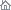Home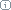About Us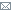ContactBlogWhat's NewProductsBuy NowDownloadsForumGeneXproTools Online Guide Learn how to use the 5 modeling platforms of GeneXproTools with the Online GuideLast update: February 19, 2014Data Normalization

GeneXproTools supports different kinds of data normalization (Standardization, 0/1 Normalization and Min/Max Normalization), normalizing all numeric input variables using data statistics derived from the training dataset. This means that the validation/test dataset is also normalized using the training data statistics such as averages, standard deviations, and min and max values evaluated for all numeric variables.

Data normalization can be very useful for datasets with variables in very different scales or ranges. Note, however, that data normalization is not a requirement even in these cases, as the learning algorithms of GeneXproTools can handle unscaled data quite well. Notwithstanding, GeneXproTools allows you to check very quickly and easily if normalizing your data improves modeling: if not, also as quickly, you can revert to the original raw data.

It’s worth pointing out that GeneXproTools offers not just a convenient way of trying out different normalization schemes. As is the case for categorical variables and missing values, GeneXproTools generates code that also supports data scaling, allowing you to deploy your models confidently knowing that you can use exactly the same data format that was used to load the data into GeneXproTools. Below is a sample code in R of a regression model created using data standardized in the GeneXproTools environment.

#------------------------------------------------------------------------
# Regression model generated by GeneXproTools 5.0
# GEP File: D:\GeneXproTools\V5.0\OnlineGuide\ConcreteStrength-Std_01.gep
# Training Records:  687
# Validation Records:   343
# Fitness Function:  Positive Correl
# Training Fitness:  902.425484649759
# Training R-square: 0.814371755345348
# Validation Fitness:   910.563337228454
# Validation R-square:  0.829125591104619
#------------------------------------------------------------------------

gepModel <- function(d)
{
G1C5 <- -9.56011932737205
G1C8 <- -8.63162785729545
G2C3 <- 1.49617681508835
G3C1 <- 2.18332468642232
G3C6 <- -2.90885921811579
G3C7 <- 1.75264748069704
G4C8 <- 1.12216559343242
G6C5 <- -3.60847804193243

d <- Standardize(d)
y <- 0.0

y <- exp(((min(((G1C8+G1C8)/2.0),(d+d))-(d*d))-G1C5))
y <- y + (d/((G2C3+d)/2.0))
y <- y + ((G3C1+(tanh(G3C7)*d))+((tanh(d)+(G3C6-d))/2.0))
y <- y + (d-(1.0-((((min(d,d)+(d*G4C8))/2.0)+((d+d)/2.0))/2.0)))
y <- y + atan(d)
y <- y + ((gep3Rt(d)+max(((d ^ 2)-(1.0-G6C5)),(d+d)))/2.0)

y <- Reverse_Standardization(y)

return (y)
}

gep3Rt <- function(x)
{
return (if (x < 0.0) (-((-x) ^ (1.0/3.0))) else (x ^ (1.0/3.0)))
}

Standardize <- function (input)
{
AVERAGE_1 <- 280.949490538574
STDEV_1 <- 102.976876719742
input <- (input - AVERAGE_1) / STDEV_1

AVERAGE_2 <- 73.3764192139738
STDEV_2 <- 85.4464915167598
input <- (input - AVERAGE_2) / STDEV_2

AVERAGE_3 <- 55.0788937409025
STDEV_3 <- 64.0807915707749
input <- (input - AVERAGE_3) / STDEV_3

AVERAGE_4 <- 181.878602620087
STDEV_4 <- 21.7339765533138
input <- (input - AVERAGE_4) / STDEV_4

AVERAGE_5 <- 6.12983988355168
STDEV_5 <- 5.93069279508886
input <- (input - AVERAGE_5) / STDEV_5

AVERAGE_6 <- 973.916593886463
STDEV_6 <- 76.777259058253
input <- (input - AVERAGE_6) / STDEV_6

AVERAGE_7 <- 771.181804949053
STDEV_7 <- 79.7070075911026
input <- (input - AVERAGE_7) / STDEV_7

AVERAGE_8 <- 45.2823871906841
STDEV_8 <- 64.9243023773916
input <- (input - AVERAGE_8) / STDEV_8

return (input)
}

Reverse_Standardization <- function(modelOutput)
{
# Model standardization
MODEL_AVERAGE <- 0.836965914165358
MODEL_STDEV <- 1.73854230290885
modelOutput <- (modelOutput - MODEL_AVERAGE)/MODEL_STDEV

# Reverse standardization
TARGET_AVERAGE <- 35.49461426492
TARGET_STDEV <- 16.3004798384353

return (modelOutput * TARGET_STDEV + TARGET_AVERAGE)
}

It’s also worth pointing out that for regression problems with a continuous response variable, the response variable is also normalized. For model deployment this also requires the reverse-normalization of the model output of the generated models, which GeneXproTools implements in all the code generated for model scoring. Note, however, that on the charts and tables for model visualization and selection within GeneXproTools, the raw “normalized” model output (not really normalized, but generated to match normalized actual values) is shown, as it is usually compared with the normalized response variable.

An interesting and useful application of this normalization/reverse-normalization technique in regression problems is that, with normalized data, the fitness functions strictly based on correlations between predicted and actual values (R-square, Bounded R-square, Positive Correl and Bounded Positive Correl), work just like any other fitness function in the sense that the model output is brought back to scale by the reverse-normalization function. This might prove advantageous for problems where higher R-square values are easier and faster to achieve with an R-square-like fitness function than with any other function. The reason for this lies in the fact that R-square-like fitness functions measure only correlation, allowing evolution to take place over a richer unconstrained fitness landscape.

Related Tutorials:

Related Videos:

 Leave Feedback Please enter the number below using the combo boxes before sending your feedback. 3 8 4 0 1 2 3 4 5 6 7 8 9   0 1 2 3 4 5 6 7 8 9   0 1 2 3 4 5 6 7 8 9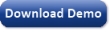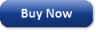Time Limited Trial

Released February 19, 2014

Last update: 5.0.3883New Entries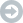Subscribe to the GEP-List

 3 8 4 0 1 2 3 4 5 6 7 8 9   0 1 2 3 4 5 6 7 8 9   0 1 2 3 4 5 6 7 8 9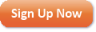Home | What's New | Products | Buy Now | Downloads | Quick Tour | Support | Contact Us | About Gepsoft | Sign UpForum | Blog | Videos | Tutorials | Server Knowledge Base | Logistic Regression Guide | Terms of Use | Privacy & Cookies